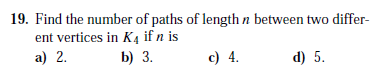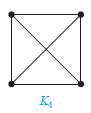This topic is 2084 days old which is more than the 365 day threshold we allow for new replies. Please post a new topic.

## Recommended PostsI am doing part a of Ex 19. The answer has 2 but I can list at least 11 paths.

Here are the paths:

1) {a,b,d}

2) {a,d,c}

3) {a,c,d}

4) {b,a,c}

5) {b,d,c}

6) {b,c,d}

7) {c,a,b}

8) {c,d,b}

9) {d,b,a}

10) {d,c,a}

11) {d,b,c}

The complete graph k4 graph does not have labeled vertices a,b,c,d.

So I am not sure if I can label a,b,c,d anywhere I want.

Edited by warnexus

##### Share on other sites

Sure you can label them as you want.

I think you are misunderstanding the problem, though. I would interpret it as, “Pick two fixed vertices. Tell me how many paths of length 2 there are between them.” The beauty here is that you should get the same answer no matter which two vertices you pick – this is thanks to your graph being a complete one; the question would be ill-formed if it were for a different kind of graph.

##### Share on other sites

Assuming the diagonals count as 1, if you pick any 2 vertices, there will be only 2 paths of length 2 between them. This is what i assume you were supposed to do.

I think I get what you saying. So the 11 paths I listed are not correct.

##### Share on other sites

Sure you can label them as you want.

I think you are misunderstanding the problem, though. I would interpret it as, “Pick two fixed vertices. Tell me how many paths of length 2 there are between them.” The beauty here is that you should get the same answer no matter which two vertices you pick – this is thanks to your graph being a complete one; the question would be ill-formed if it were for a different kind of graph.

Well there are 2 edges between any two vertices you pick so 2 paths of length. Now that I am doing part b. I do not get the same answer as the textbook so I think I am struggling with what the question is actually saying

##### Share on other sites

I count

a) 2

b) 7

c) 20

d) 61

That's assuming that it's OK to repeat nodes and edges in the paths: I am not sure what precise definition you are using.

If you want more terms,

A(0) = 0, A(1) = 1, A(n) = 3*A(n-2) + 2*A(n-1) if n>1

Edited by Álvaro

##### Share on other sites

I count

a) 2

b) 7

c) 20

d) 61

That's assuming that it's OK to repeat nodes and edges in the paths: I am not sure what precise definition you are using.

If you want more terms,

A(0) = 0, A(1) = 1, A(n) = 3*A(n-2) + 2*A(n-1) if n>1

You did get them all correct. You mention you counting the edges, so you do not need to write them down on a piece of paper?

Can you explain how you get 7 for part b?

I think if I understand how 7 was acheived I should be able to get part c and d intuitively. part a seems to do a poor job of figuring out how the thought process should be approached.

Edited by warnexus

##### Share on other sites

A(0) = 0, A(1) = 1, A(n) = 3*A(n-2) + 2*A(n-1) if n>1

To find a path with length n you need to find a path with length n-1 to any of the other nodes. You have 2 options:
1. Find a path to a node other than the start node. So 2 possible nodes, and you need a path of length n-1 to get to them. This is the 2*A(n-1) term.

2. Find a n-1 path to the start node. To do that, you need a n-2 path to any of the other 3 nodes. That's the 3*A(n-2) term.

##### Share on other sites

A(0) = 0, A(1) = 1, A(n) = 3*A(n-2) + 2*A(n-1) if n>1

To find a path with length n you need to find a path with length n-1 to any of the other nodes. You have 2 options:
1. Find a path to a node other than the start node. So 2 possible nodes, and you need a path of length n-1 to get to them. This is the 2*A(n-1) term.

2. Find a n-1 path to the start node. To do that, you need a n-2 path to any of the other 3 nodes. That's the 3*A(n-2) term.

Oh I get it now. I was doing it wrong the whole time. Thanks for the explaination.

• 9
• 17
• 10
• 11
• 18